Author: Oscar Cronquist Article last updated on October 15, 2018

In this article I will demonstrate two techniques for counting per row. The first example is simple and straightforward. The second example is a bit more complicated but dynamic and automatic, you only need to provide a search string, everything else is calculated by array formulas.

The first example has this data table.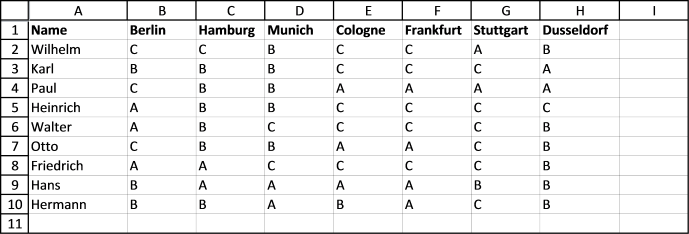I want to count the value "C" per row. In cell I1, type: C.
In cell I2, type:

=COUNTIF(B2:H2,\$I\$1)

Copy cell I2 and paste to I3:I10.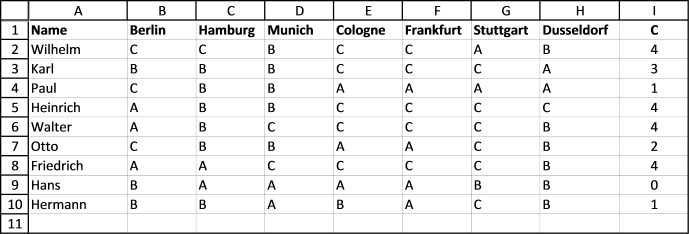The COUNTIF function counts the number of cells that meet a given condition.

=COUNTIF(range, criteria)

There are 4 C's in row 2 so the formula returns 4 in cell I2. If you change the value in cell I1 to "B", the formulas in I2:I10 recalculates and return new values.

Let us sort the table. Select cell range A1:I10. Go to tab "Home" on the ribbon. Click "Sort & Filter" button. Click "Filter".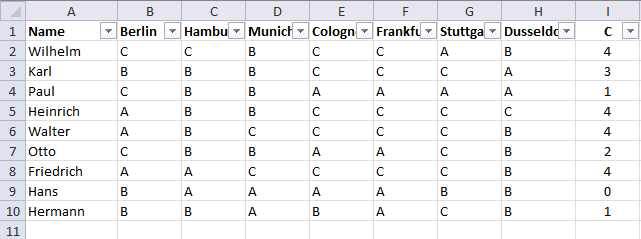Drop down list arrows appear next to each header, see picture above. Click the arrow next to "C".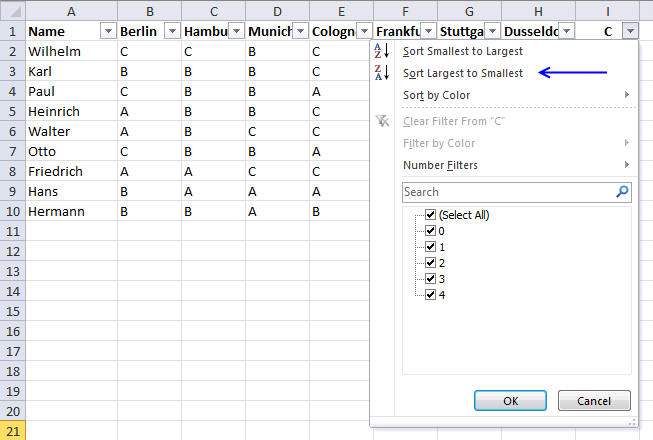Click "Largest to smallest". The data table shows the count of "C" per row, sorted largest to smallest.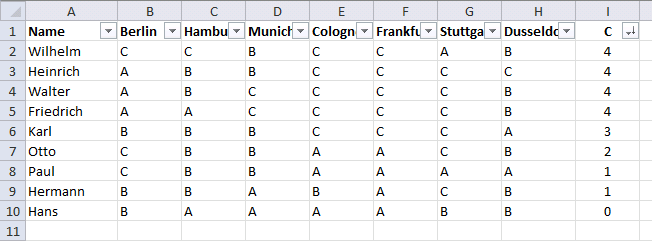Do you know why \$I\$1 in COUNTIF(B2:H2,\$I\$1) has dollar signs? Read this post:
Absolute and relative cell references

### Dynamic counting

Now on to a more interesting and complicated example. The formula here returns names sorted in column D based on the number of "C"s per row.

Change the value in cell B3 and the list in cell range D3:E11 is instantly changed.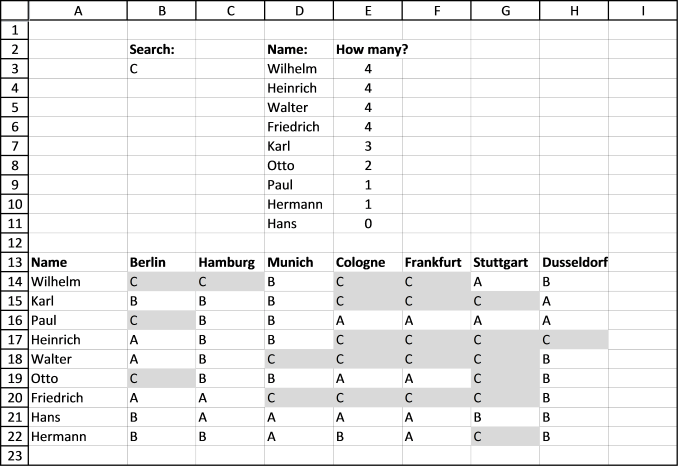I have applied conditional formatting to cell range A14:H22 so you can easily verify the calculated numbers in E3:E11.

Array formula in cell D3:

=INDEX(\$A\$14:\$A\$22, MATCH(LARGE(MMULT((\$B\$14:\$H\$22=\$B\$3)*1, TRANSPOSE(COLUMN(\$B\$14:\$H\$22)^0)), ROWS(\$A\$1:A1)), IF(COUNTIF(\$D\$2:\$D2, \$A\$14:\$A\$22)=0, MMULT((\$B\$14:\$H\$22=\$B\$3)*1, TRANSPOSE(COLUMN(\$B\$14:\$H\$22)^0)), ""), 0))

Array formula in cell E3:

=LARGE(MMULT((\$B\$14:\$H\$22=\$B\$3)*1, TRANSPOSE(COLUMN(\$B\$14:\$H\$22)^0)), ROWS(\$A\$1:A1))

Functions in this formula: INDEX, MATCH, LARGE, MMULT, ROW COUNTIF, IF

Interested in learning more about excel formulas? Check out the Advanced excel course.